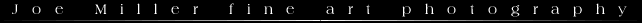NOTE:  In the near future I may discoutinue some of the javascript calculators on this site.  If you are interested in downloading a free border caculator go to http://www.fineart-photography.com/BorderCalc.html

*   *   *

Somewhere I once saw a diagram  showing how to place an image in the (so called) "optical center" of a frame.  I converted this diagram into a formula and constructed the javascript table below that will give you the border widths for cutting a window mat.  Using the measurements provided will result in the image being closer to the top of the frame than the bottom.   The top and bottom borders are relative to the width of the image or window.   For example, a horizontal 8 x 10 will be slightly higher in the frame than a horizontal 8 x 12.

Since some of us insist on using inches, I have provided a table to covert inches into a decimal fraction that can be entered into the Optical Center table.  Below the table, there is a javascript program to convert back to inches.  I figured the nearest one-sixteenth of an inch was close enough for most purposes and that everyone would know that two sixteenths is one-eight and so on.  Use this at your own risk.  If you have questions, problems or comments feel free to email me.1/16 = .0625 5/16 = .3125 9/16 = .5625 13/16 = .8125 2/16 or 1/8 = .1250 6/16 or 3/8 = .3750 10/16 or 5/8 = .6250 14/16 or 7/8 = .8750 3/16 = .1875 7/16 = .4375 11/16 = .6875 15/16 = .9375 4/16 or 1/4 = .2500 8/16 or 1/2 = .5000 12/16 or 3/4 = .7500
 Length of Mat Width of Mat Length of Window Width of Window Optical Center Adjustment Top Border Bottom Border Side Borders Messages

Convert Decimal Fraction to Nearest Sixteenth of an Inch

Decimal Fraction:    would be:  sixteenths*
*CAUTION:  Use the above converter for either the top or bottom border and find the other by subtracting the found border and window from the length of the mat.  Since the converter rounds, if both the top and bottom were rounded in the same direction, the result could be 1/16 of an inch off.

Matting Prints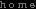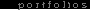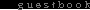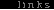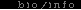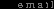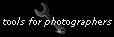All Text and Images © Joe Miller, 2004